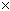SEARCH HOMEMath Central Quandaries & QueriesQuestion from jennifer: assume that y varies directly as x. find y when x = 54 if y =1/4 when x=3/2Hi Jennifer.

When we say y varies directly as x, we mean that the ratio between these variables is constant. In fact, if we write the ratio as a fraction (y / x), then we call this fraction the constant of variation. So any fraction that equals this constant is a value pair that satisfies the conditions.

Here is an example. Assume that y varies directly as x. Find y when x = 10 if y = 7.5 when x = 3.

This means we know the constant of variation is 7.5 / 3, which equals 2.5. So any fraction that equals 2.5 satisfies the conditions. Therefore if y / x = 2.5 and x = 10, then y / 10 = 2.5, so y = 2.510 = 25.

Your question can be answered using exactly the same technique Jennifer.

Hope this helps,
Stephen La Rocque.Math Central is supported by the University of Regina and The Pacific Institute for the Mathematical Sciences.